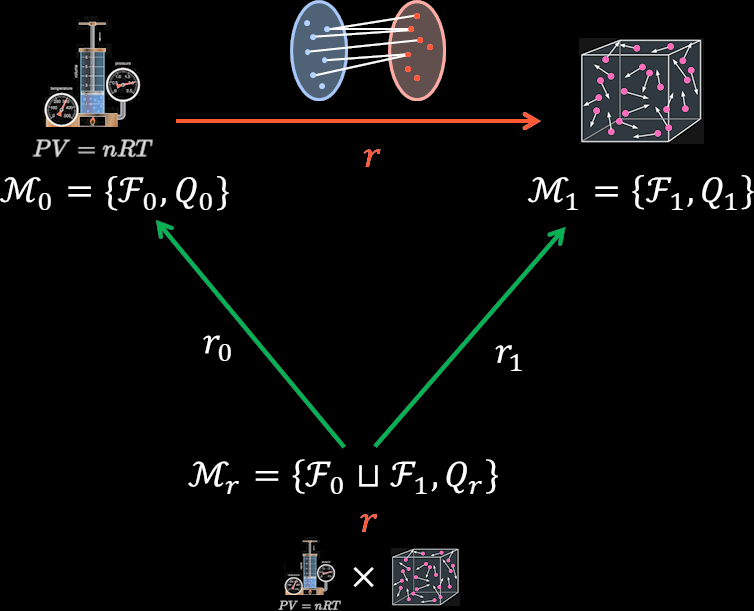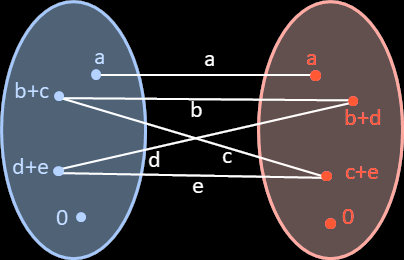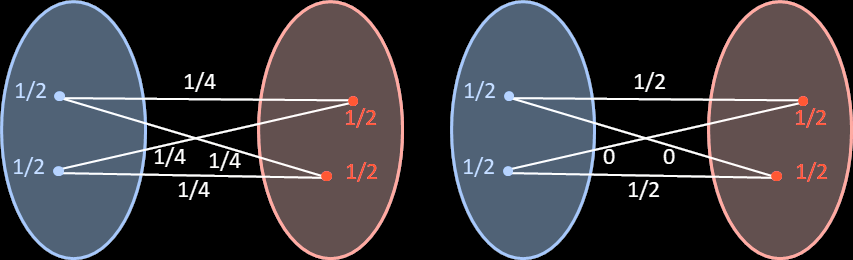# The underlying model of a morphism

I’ve already talked about generalised models. The aim is not only to have a universal system for modelling any agent’s mental model—universality is pretty easy to get—but a system where it’s easy to recreate these mental models. And then analyse the transition between models.

This post will show that if there is a morphism between two models (say, between ideal gas laws and models of atoms bouncing around), then there is an underlying model for that morphism.

Specifically, if is a morphism between and , then there is a generalised model defined from . The features of this model are the combination of the features of the two models: , and there are natural morphisms and from this underlying model to and :Now, if and are the sets of possible worlds for and , then is the set of possible worlds for . Then since is a relation between and , it can be seen as subset of . And the is a probability distribution over this subset .

What this means is that measures how probability ‘flows’ from worlds in to worlds in . If is an element of , then measures how much probability is flowing from to . The actual probability of is the sum of all probability flowing out of it; that of , the sum of the probability flowing into it.

See for example this diagram, where the probabilities are indicated in blue, those of in black, and those of in red. The probabilities and are the sum of the relevant probabilities on the “edges” connecting to those points:The distribution is non-unique, though. The following two examples show situations with the same and , but different :The rest of this post will be dedicated to prove the existence of the underlying model for the morphism ; it can be skipped if you aren’t interested.

# Proof of underlying model

## Definitions

Previous posts on generalised models defined them as triplets , with a set of features, the set of possible worlds for those features, a subset of environments, and a probability distribution on .

But was mainly superfluous, as can be extended to a probability distribution on all of just by setting it to be zero on . Thus was dropped from the definition.

The original definition allowed to be a partial probability distribution, but here we’ll assume it’s a total probability distribution (though not necessarily normalised; need not be ). The sets of features are assumed to be finite.

Then a morphism between generalised models and is a binary relation between and , such that:

1. ,

2. .

We might extend the class of morphisms by defining relations that only obey the first inequality as “left-morphisms”, and relations that only obey the second one as a “right-morphisms”. Left-morphisms ensure probability isn’t lost (), right morphisms ensure probability isn’t gained (). Full morphisms, of course, ensure that probability isn’t gained or lost ().

Binary relations are not necessarily functions; functions are relations such that each in is related to exactly one in .

## Statement of the theorem

Let be a morphism between and . Then there exists a generalised model , with natural function morphisms and .

The is non-zero on a set contained in . The need not be uniquely defined, but the total measure of is the same as and :

## Main proof

The function is just projection onto the first component: it sends to . The functions conversely send to .

Because and are functions, they can ‘push-forward’ any probability distribution on to and , respectively. This is given by: , and similarly for .

We aim to construct a such that and ; this will be our , and will make and into morphisms.

Define to be zero if , or or . Thus we will ignore any elements of and of measure zero, and any element of that is not in .

Let be such that it is not related to any elements of by . Then . Thus any element of with non-zero measure is related to some via .

Then define a choice function that maps every element with , to an element that it is related to by . And define , and is zero on all other elements of .

Then . Hence . Consequently, .

Define as the set of , probability distributions on with . We’ve shown that is non-empty; moreover, any has a total measure equal to . Since is defined on , then it is contained in the set .

The set is compact, and is a closed condition, so is compact. The next section will prove that there is an element with ; that will complete the proof.

## Key lemmas

Define . Now , and note that is equivalent with .

Thus if takes the value on , we’ve found the desired . We will show that this happens thanks to the following key lemma:

• Lemma 1: If there is a with , then there exists a with .

Now, since is compact and is continuous, it will attain its minimum on . Then lemma 1 shows that (otherwise it wouldn’t be a minimum).

Proof of Lemma 1:

Fix a with . Now . So, since , there must exist a with .

By lemma 2 (see below), we’ll show that there exists a path with the following properties:

1. ,

2. and are both elements of ,

3. the are all greater than ,

4. is such that .

Then define to be the minimum of .

We’ll then define as (which is greater than by the definition of ), , and otherwise.

Then notice that, apart from and , . So and differ only on and ; specifically

• ,

• .

Since and , we have . This proves Lemma 1.

• Lemma 2: There exists a path with the following properties:

1. ,

2. and are both elements of ,

3. the are all greater than ,

4. is such that .

Proof of Lemma 2:

Let be the set of all elements of that can be reached by paths (ie are ) that obey the first three properties above. Let be the set of all elements of that are for some path that obey the first three properties above. Then clearly , by the second condition above (note that the third condition doesn’t affect , which is only required to be in ).

Since is a morphism, .

Note that if with , then must be in ; this is because we could add as to any path that reaches , getting a slightly longer path that goes via and thus puts it in .

Consequently, .

So . Since includes with , it also much include at least one with .

The path that reaches this will then satisfy the fourth condition of the lemma, proving it.

No comments.